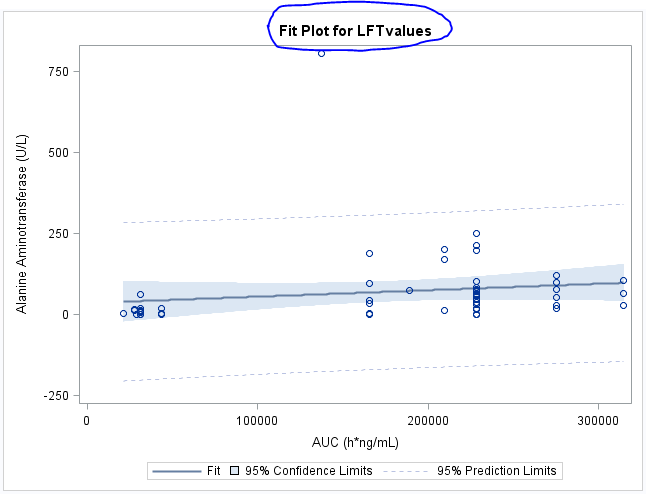## Regression Plot with Confidence 95% CI Bounds

Hello!

I need some assistance.  A simple linear regression script such as the following would give me a regression plot with 95% CI bounds on defaultRegression Plot.

Proc glm data= Tdata;
model LFTvalues = bckTrans_auc/ solution;
run;

Please how can I use Proc SGplot to replicate exact same plot and have full control of things display like removing the title from the plot?
1 ACCEPTED SOLUTION

Accepted Solutions

## Re: Regression Plot with Confidence 95% CI Bounds

Already figured out a way to solve my problem

proc sgplot data= Tdata;

reg x=bckTrans_auc y=LFTvalues/CLM CLI;

yaxis label = "AST (Units per liter)";
xaxis label = "AUC(h*ng/mL)";

Run;

4 REPLIES 4

## Re: Regression Plot with Confidence 95% CI Bounds

Thanks @Reeza

The following codes solved my problem

proc sgplot data= Tdata; reg x= bckTrans_auc y=LFTvalues/CLM CLI; yaxis label = "AST (Units per liter)"; xaxis label = "AUC(h*ng/mL)"; Run;

Please do you know how I can include the regression equation on the plot?

## Re: Regression Plot with Confidence 95% CI Bounds

AFAIK there isn't an option for that but you can check the INSET statement or REG statement options which is where I'd expect to see it.
Otherwise, here's an alternative method.
https://blogs.sas.com/content/iml/2013/02/27/slope-of-a-regression-line.html

## Re: Regression Plot with Confidence 95% CI Bounds

Already figured out a way to solve my problem

proc sgplot data= Tdata;

reg x=bckTrans_auc y=LFTvalues/CLM CLI;

yaxis label = "AST (Units per liter)";
xaxis label = "AUC(h*ng/mL)";

Run;

Discussion stats
• 4 replies
• 783 views
• 5 likes
• 2 in conversation